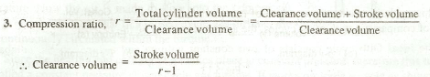### Otto Cycle

Otto Cycle was originally devised by a Frenchman Beau-de-Rochas in 1862. The first successful engine, working on this cycle, was built by a German engineer Nicholas A. Otto in 1876. These days, many Gas, Petrol and many of the oil engines run on this cycle. It is also known as constant volume cycle, as the heat is received and rejected at a constant volume.

This cycle is taken as a standard of comparison for internal combustion engines. For the purpose of comparison with other cycles, the air is assumed to be the working substance.

The ideal Otto cycle consists of two constant volume and two reversible adiabatic or isentropic processes as shown on p-v and T-s diagrams in Fig. 5.11 (a) and (b) respectively.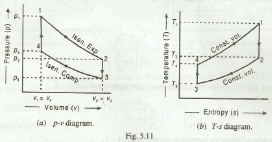The efficiency of the Otto cycle (also known as ideal efficiency or air standard efficiency) is given by equation no (i):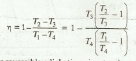We know that for reversible adiabatic or isentropic expansion process 1-2,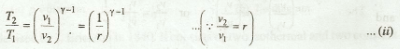and for reversible adiabatic or isentropic compression process 3 - 4,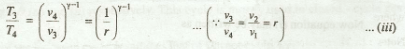From equations (ii) and (iii),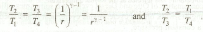Now equation (i) may be written as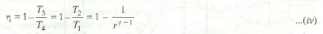Notes:

1. We see from equation (iv) that the efficiency of the Otto cycle depends on compression ratio (r).
2. The efficiency increases with the compression ratio (r). In actual practice, r can not be increased beyond a value of 7 or so.